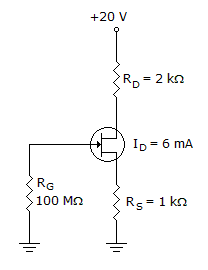# Electronic Devices - Field-Effect Transistors

Exercise : Field-Effect Transistors - General Questions
1.
For a JFET, the value of VDS at which ID becomes essentially constant is the
pinch-off voltage.
cutoff voltage.
breakdown voltage.
ohmic voltage.
Explanation:
No answer description is available. Let's discuss.

2.
The ________ has a physical channel between the drain and source.
D-MOSFET
E-MOSFET
V-MOSFET
Explanation:
No answer description is available. Let's discuss.

3.

Refer to figure given below. Calculate the value of VDS.0 V
2 V
4 V
–2 V
Explanation:
No answer description is available. Let's discuss.

4.

Refer to figure shown below. Determine the value of VS.20 V
8 V
6 V
2 V